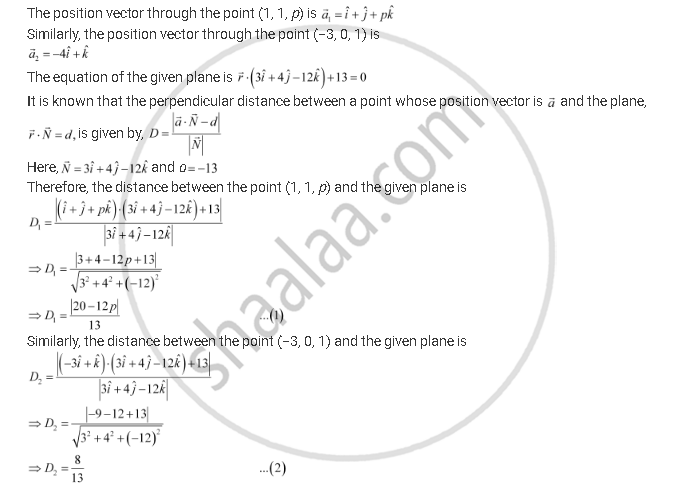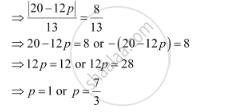Share

# If the Points (1, 1, P) and (−3, 0, 1) Be Equidistant from the Plane Vecr.(3hati + 4hatj - 12hatk)+ 13 = 0, Then Find the Value Of P. - CBSE (Science) Class 12 - Mathematics

ConceptPlane Equation of a Plane Perpendicular to a Given Vector and Passing Through a Given Point

#### Question

If the points (1, 1, p) and (−3, 0, 1) be equidistant from the plane vecr.(3hati + 4hatj - 12hatk)+ 13 = 0, then find the value of p.

#### SolutionIt is given that the distance between the required plane and the points, (1, 1, p) and (−3, 0, 1), is equal.

∴ D1 = D2Is there an error in this question or solution?

#### APPEARS IN

NCERT Solution for Mathematics Textbook for Class 12 (2018 to Current)
Chapter 11: Three Dimensional Geometry
Q: 14 | Page no. 498

#### Video TutorialsVIEW ALL 

Solution If the Points (1, 1, P) and (−3, 0, 1) Be Equidistant from the Plane Vecr.(3hati + 4hatj - 12hatk)+ 13 = 0, Then Find the Value Of P. Concept: Plane - Equation of a Plane Perpendicular to a Given Vector and Passing Through a Given Point.
S